Question

step by step. box in answer please Rigid rods of negligible mass lying along the y...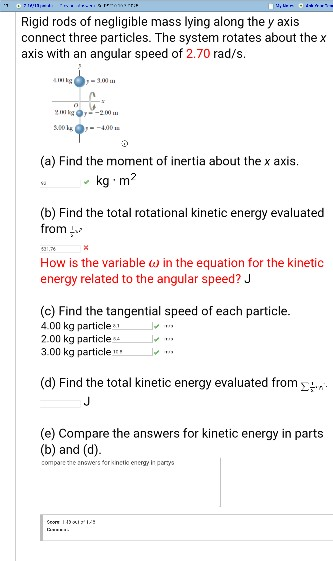Rigid rods of negligible mass lying along the y axis connect three particles. The system rotates about the x axis with an angular speed of 2.70 rad/s (a) Find the moment of inertia about the x axis kg m (b) Find the total rotational kinetic energy evaluated from How is the variable ω in the equation for the kinetic energy related to the angular speed? J (c) Find the tangential speed of each particle. 4.00 kg particle:i 2.00 kg particle , 3.00 kg particle (d) Find the total kinetic energy evaluated from- (e) Compare the answers for kinetic energy in parts (b) and (d) ompo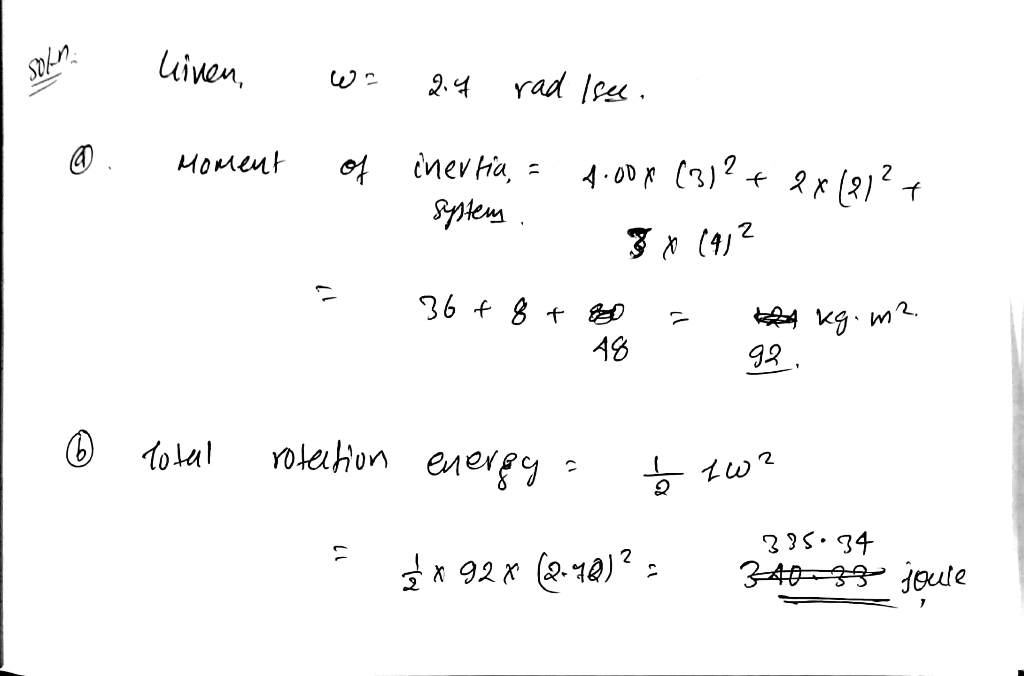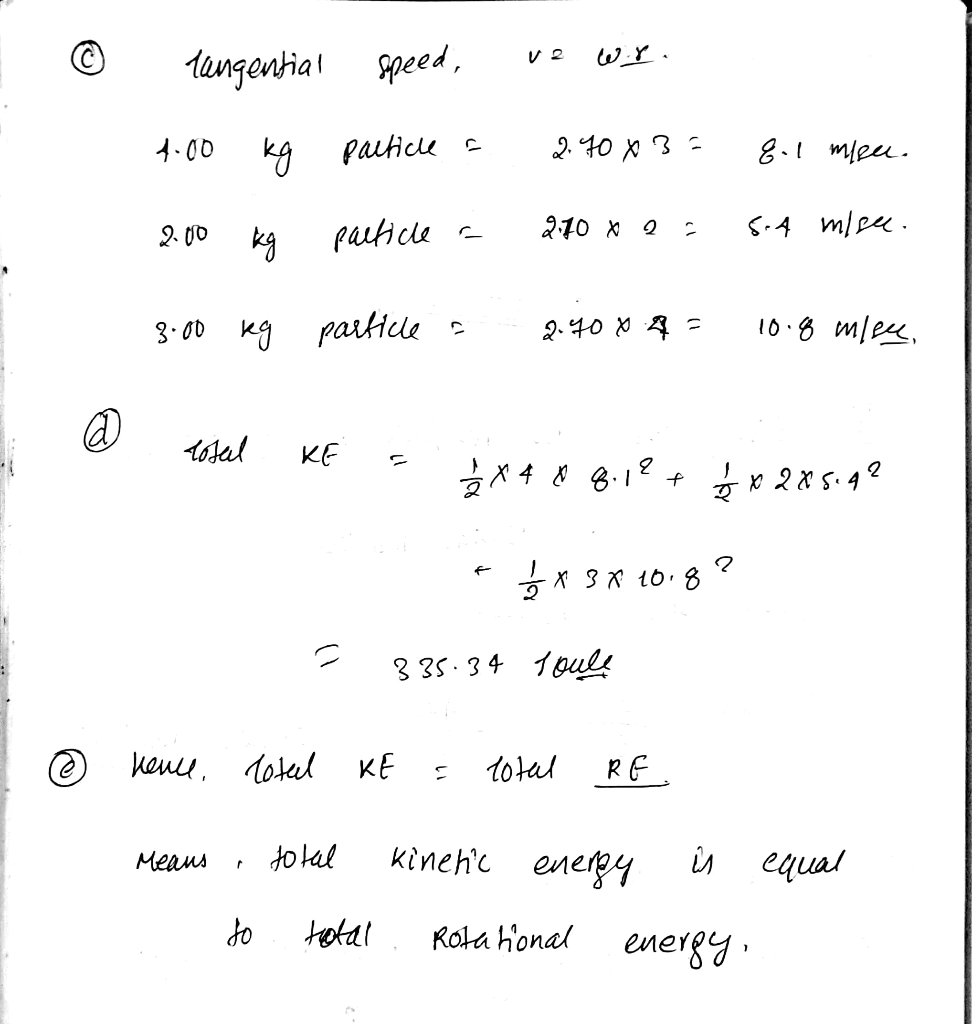Earn Coins

Coins can be redeemed for fabulous gifts.

Similar Homework Help Questions
• rods of negligible mass lying along the y axis connect three particles. The system rotates about...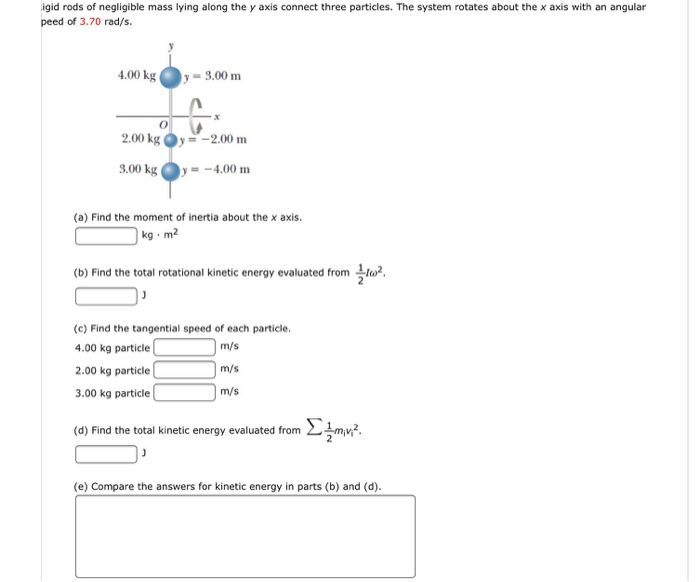rods of negligible mass lying along the y axis connect three particles. The system rotates about the x axis with an angular peed of 3.70 rad/s. (a) Find the moment of inertia about the x axis. kg middot m^2 (b) Find the total rotational kinetic energy evaluated from 1/2 I omega^2. J (c) Find the tangential speed of each particle. 4.00 kg particle m/s 2.00 kg particle m/s 3.00 kg particle m/s (d) Find the total kinetic energy evaluated from...

• Rigid rods of negligible mass lying along the y axis connect three particles. The system rotates...

Rigid rods of negligible mass lying along the y axis connect three particles. The system rotates about the x axis with anangular speed of 1.50 rad/s.(b) Find the tangential speed of each particle.m/s (4.00 kg particle)m/s (2.00 kg particle)m/s (3.00 kg particle)

• Rigid rods of negligible mass lying along the y axis connect three particles. The system rotates...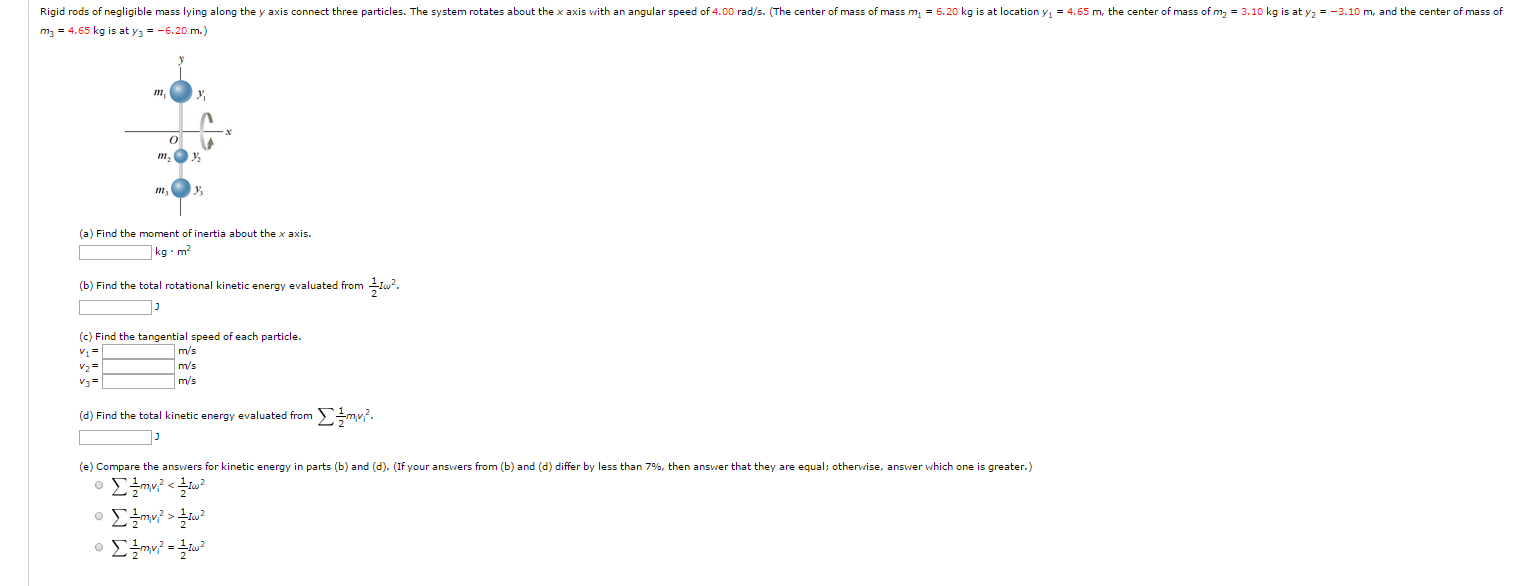Rigid rods of negligible mass lying along the y axis connect three particles. The system rotates about the x axis with an angular speed of 4.00 rad/s. (The center of mass of mass m1 = 6.20 kg is at location yl = 4.65 m, the center of mass of m2 = 3.10 kg is at y2 = -3.10 m, and the center of mass of m2 = 4.65 kg is at y3 = -6.20 m.)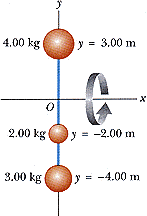Rigid rods of negligible mass lying along the y axisconnect three particles (Fig. P10.20). The system rotates about thex axis with an angular speedof 3.00 rad/s.Figure P10.20(a) Find the moment of inertia about thex axis.kg·m2Find the total rotational kinetic energy evaluated from 1/2I2. J(b) Find the tangential speed of each particle. m/s (4.00 kgparticle) m/s (2.00 kgparticle) m/s (3.00 kgparticle)Find the total kinetic energy evaluated from mivi2/2. J

• The four particles shown below are connected by rigid rods of negligible mass where y1 = 6.60 m.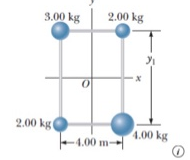The four particles shown below are connected by rigid rods of negligible mass where y1 = 6.60 m. The origin is at the center of the rectangle. The system rotates in the xy plane about the z axis with an angular speed of 6.40 rad/s. (a) Calculate the moment of inertia of the system about the z axis.(b) Calculate the rotational kinetic energy of the system.

• The four particles shown below are connected by rigid rods of negligible mass where y1 = 6.60 m.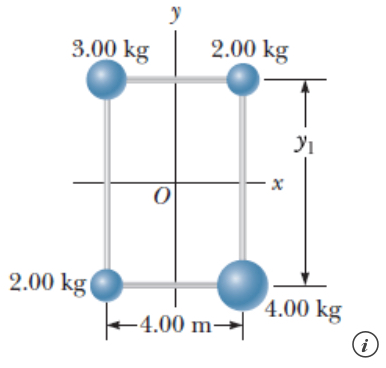The four particles shown below are connected by rigid rods of negligible mass where y1 = 6.60 m. The origin is at the center of the rectangle. The system rotates in the xy plane about the z axis with an angular speed of 5.40 rad/s. (a) Calculate the moment of inertia of the system about the z axis.(b) Calculate the rotational kinetic energy of the system.

• The four particles shown below are connected by rigid rods of negligible mass where y1 = 6.60 m

The four particles shown below are connected by rigid rods of negligible mass where y1 = 6.60 m. The origin isat the center of the rectangle. The system rotates in the xy plane about the z axis with an angular speed of 5.70rad/s.(b) Calculate the rotational kinetic energy of the system.

• The figure shows three particles connected on a rod, of negligible mass, along the y axis....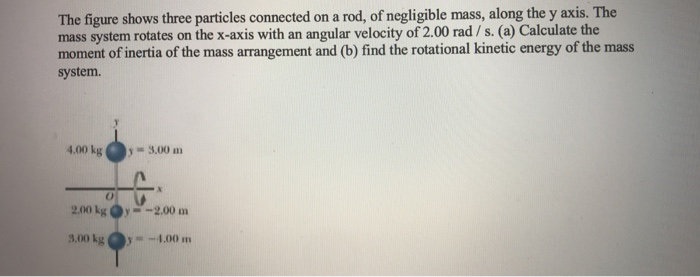The figure shows three particles connected on a rod, of negligible mass, along the y axis. The mass system rotates on the x-axis with an angular velocity of 2.00 rad / s. (a) Calculate the moment of inertia of the mass arrangement and (b) find the rotational kinetic energy of the mass system 4.00 kg , -9.00 m 2004 -2,00 m 3,00 kg y -4.00 m

• Four particles with masses 4 kg, 6 kg, 4 kg, and 6 kg are connected by rigid rods of negligible mass as shown.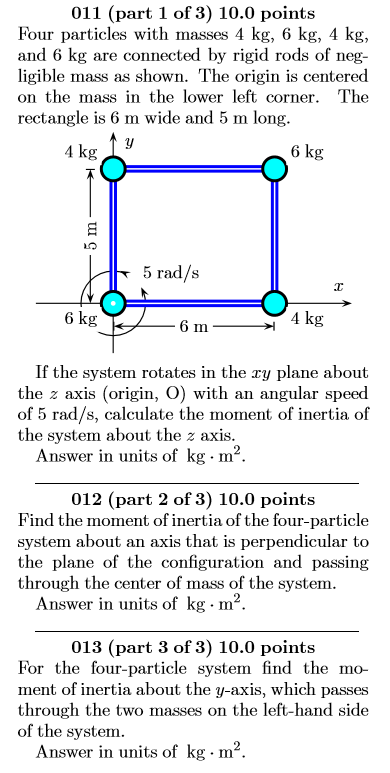011. Four particles with masses 4 kg, 6 kg, 4 kg, and 6 kg are connected by rigid rods of negligible mass as shown. The origin is centered on the mass in the lower left corner. The rectangle is 6 m wide and 5 m long. If the system rotates in the xy plane about the z axis (origin, O) with an angular speed of 5 rad/s, calculate the moment of inertia of the system about the z axis. 012. Find the...

• The four particles shown in Fig. conriected by rigid rods. The origin is at the center...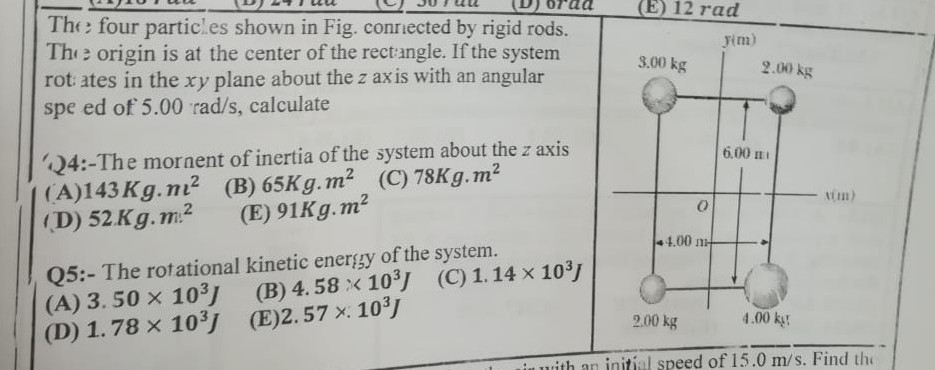The four particles shown in Fig. conriected by rigid rods. The origin is at the center of the rectangle. If the system rot ates in the xy plane about the z axis with an angular spe ed of 5.00 rad/s, calculate Fim) 3.00 kg 2.00 kg 4:-The mornent of inertia of the system about the z axis (A)143 Kg ni2 (B) 65Kg.m2 (C) 78Kg.m2 (D) 52 Kg.m2 (E) 91Kg.m2 6.00 ni im) 4.00mH Q5:- The rotational kinetic energy of the...